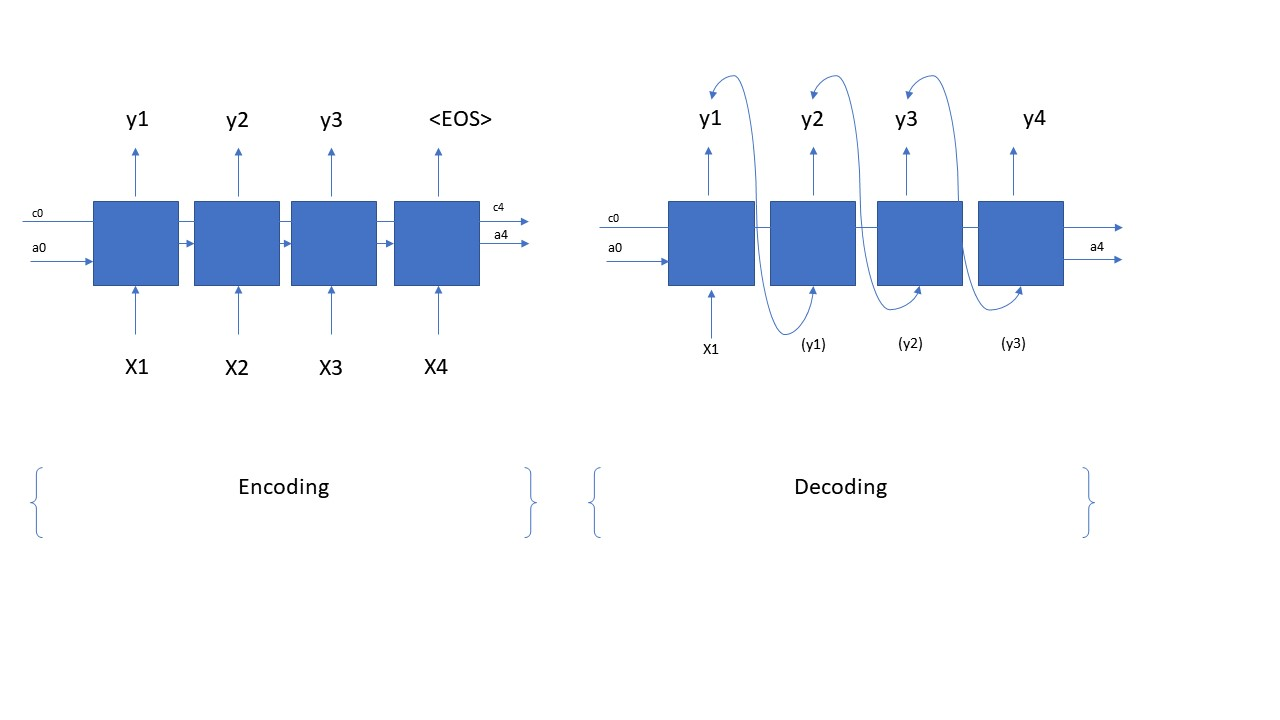# 机器学习 - LSTM应用之sequence generation

2020/03/10 22:47

• 概述

LSTM在机器学习上面的应用是非常广泛的，从股票分析，机器翻译 到 语义分析等等各个方面都有它的用武之地，经过前面的对于LSTM结构的分析，这一节主要介绍一些LSTM的一个小应用，那就是sequence generation。其实sequence generation本事也是对一些应用的统称，例如: 让机器学习音乐后然后让机器根据学习的模型自己创造音乐（制作人快要失业啦。。。。），让机器学习某种语言然后让这个学习到的模型自己产生Word来说话，等等。这其实本质是一种one-to-many的LSTM网络结构。这一节内容主要就是讲解这一种网络结构的应用。

• Sequence generation的网络结构分析• Sequence generation 代码分析

从上面的分析，咱们可以看出sequence generation是由两个部分组成，那么自然咱们代码也肯定得分成两部分来实现上图中的网络结构，那么接下来咱们来看看第一步，就是如何用Python来实现encoding的结构，代码如下所示，咱们看着代码来慢慢分析：

#define shared variablesn_a=64
n_values = 78 # dimensions of out single input
reshapor = keras.layers.Reshape((1, n_values))                  # Used in Step 2.B of djmodel(), below
LSTM_cell = keras.layers.LSTM(n_a, return_state = True)         # Used in Step 2.C, return_state muset be set
densor = keras.layers.Dense(n_values, activation='softmax')     # Used in Step 2.D
#multiple inputs (X, a, c), we have to use functional Keras, other than sequential APIs
def create_model(Tx, n_a, n_values):
"""
Implement the model

Arguments:
Tx -- length of the sequence in a corpus
n_a -- the number of activations used in our model
n_values -- number of unique values in the music data

Returns:
model -- a keras instance model with n_a activations
"""

# Define the input layer and specify the shape
X = keras.Input(shape=(Tx, n_values))#input omit the batch_size dimension,  X is still 3 dimensiones (with batch_size dimension).

# Define the initial hidden state a0 and initial cell state c0
a0 = keras.Input(shape=(n_a,), name='a0')
c0 = keras.Input(shape=(n_a,), name='c0')
a = a0 c = c0 # Step 1: Create empty list to append the outputs while you iterate outputs = [] # Step 2: Loop for t in range(Tx): # Step 2.A: select the "t"th time step vector from X. x = keras.layers.Lambda(lambda x: X[:,t,:])(X) # Step 2.B: Use reshapor to reshape x to be (1, n_values) (≈1 line) #因为LSTM layer默认的输入的dimension是 (batch_size, Tx, n_values)，其中batch_size是省略的， 即是（Tx, n_values）。如果是（Tx,n_values）的话，LSTM()会默认循环Tx次，因而，咱们将它reshape成（1，n_values）,它就不会循环了。 x = reshapor(x) # Step 2.C: Perform one step of the LSTM_cell a, _, c = LSTM_cell(x, initial_state=[a,c]) # Step 2.D: Apply densor to the hidden state output of LSTM_Cell out = densor(a) #out's shape is (m,1,n_values) # Step 2.E: add the output to "outputs"  outputs.append(out) # Step 3: Create model instance model = keras.Model(inputs=[X,a0,c0],outputs=outputs) return model

def sequence_inference_model(LSTM_cell, n_values = 78, n_a = 64, Ty = 100):
"""
Uses the trained "LSTM_cell" and "densor" from model() to generate a sequence of values.

Arguments:
LSTM_cell -- the trained "LSTM_cell" from model(), Keras layer object
densor -- the trained "densor" from model(), Keras layer object
n_values -- integer, number of unique values
n_a -- number of units in the LSTM_cell
Ty -- integer, number of time steps to generate

Returns:
inference_model -- Keras model instance
"""

# Define the input of your model with a shape (it is a one-to-many structure, the input shape is (1,n_values))
x0 = keras.Input(shape=(1, n_values))

# Define a0, c0, initial hidden state for the decoder LSTM
a0 = keras.Input(shape=(n_a,), name='a0')
c0 = keras.Input(shape=(n_a,), name='c0')
a = a0 c = c0 x = x0 # Step 1: Create an empty list of "outputs" to later store your predicted values (≈1 line) outputs = [] # Step 2: Loop over Ty and generate a value at every time step for t in range(Ty): # Step 2.A: Perform one step of LSTM_cell a, _, c = LSTM_cell(x, initial_state=[a, c]) # Step 2.B: Apply Dense layer to the hidden state output of the LSTM_cell out = densor(a) # Step 2.C: Append the prediction "out" to "outputs". out.shape = (None, 78)  outputs.append(out) # Step 2.D: Select the next value according to "out", and set "x" to be the one-hot representation of the # selected value, which will be passed as the input to LSTM_cell on the next step. We have provided # the line of code you need to do this. x = keras.layers.Lambda(one_hot)(out) # Step 3: Create model instance with the correct "inputs" and "outputs" inference_model = keras.Model(inputs=[x0, a0, c0], outputs=outputs) return inference_model

inference_model = sequence_inference_model(LSTM_cell, densor, n_values = 78, n_a = 64, Ty = 50)
inference_model.summary()

x_initializer = np.zeros((1, 1, 78))
a_initializer = np.zeros((1, n_a))
c_initializer = np.zeros((1, n_a))

pred = inference_model.predict([x_initializer, a_initializer, c_initializer])

• 总结### 作者的其它热门文章

0
0 收藏

0 评论
0 收藏
0